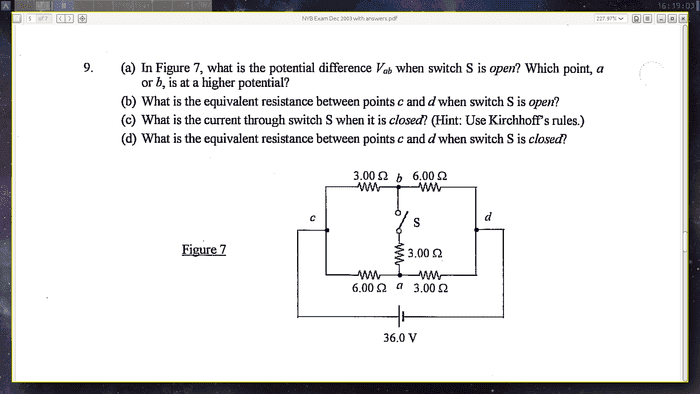# Tough Circuit Problem, Find Current When Switch is Closed

• Engineering

## Homework StatementI got questions a) and b), but I'm stuck at c) and d).

## Homework Equations

Kirchhoff's Loop and Junction rules.
Equivalent resistance in series: Req = R1 + R2
Equivalent resistance in parallel: Req = ((R1)-1 + (R2)-1)-1

## The Attempt at a Solution

I really haven't gotten anywhere on question d), I cannot figure out how to reduce it and I can't tell which resistors are in series or parallel.

My procedure for question c) started with defining multiple currents: I had I1 going to the right through the 3 ohm resistor in the top left, I2 going to the right through the 6 ohm resistor on the left, I3 going downward through the switch (and the 3 ohm resistor underneath it). I also figured that the currents going through the 6 ohm and 3 ohm resistors on the right must be I1 and I2, because the wires that are directly connected to the battery should have the same current, and you can see that current splitting into I1 and I2 on the left. Since the resistors are symmetrical, I think on the right the current must split into I1 and I2 as well.
I then used the junction rule to find that I3 = I1 + I2, from the junction on the top in the middle.
Next, I made two loop equations, and this is where I really encounter a problem:
If you take the two symmetrical loops, like one loop being the loop on the left of the switch and the other being the loop on the right of the switch, you have the same amount of equations as unkowns, because you can write I3 in terms of I1 and I2. However, both loop equations will be written entirely in terms of unknown currents, which therefore makes a homogeneous linear system in which the only system is I1 = I2 = I3 = 0.
I realized that this occurs independent of the values of the resistors and currents, so I took the loop that goes from the battery to the bottom 6 ohm resistor, to the bottom 3 ohm resistor, and back to the battery.
The loop equation, going clockwise, is:
36 - 6I2 + 3I1 = 0
Then, taking the top left loop as described above, going clockwise, I get:
-3I1 - 3(I1 + I2) + 6I2 = 0
Which reduces to:
-6I1 + 3I2 = 0
Solving both loops simultaneously, I get:
I1 = 4A
I2 = 8A
I3 = 12A

However, the right answer is I3 = 1.71A
The answer for d) is 4.21 ohms.

STEMucator
Homework Helper
Just so we're on the same page:

For a) when the switch is open, ##36.0 V## must drop across both parallel branches. You can use voltage dividers to obtain the voltage across the left hand ##3.00 \Omega## and ##6.00 \Omega## resistors. Then linking b to a with a voltage arrow and applying KVL will give ##V_{ab}## where ##a## is at a higher potential.

For b), it's simply series, series then parallel.

For c) when the switch closes, I would recommend defining two loop currents ##i_1, i_2## and all of the polarities on the resistors. Don't forget the current through the middle resistor is actually ##i_1 - i_2##. Then solve for the loop currents using mesh analysis.

For d), are you familiar with the ##\Delta - Y## transform? It would be pretty useful here.

NascentOxygen
Staff Emeritus
•